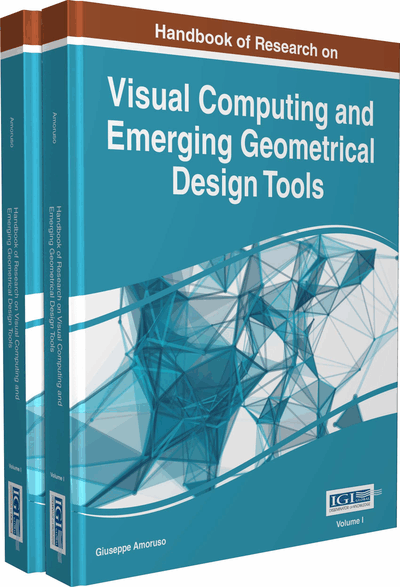# Algorithms for Geometrical Models in Borromini's San Carlino alle Quattro Fontane

Corrado Falcolini (Università degli Studi Roma Tre, Italy)
DOI: 10.4018/978-1-5225-0029-2.ch026
Available
\$37.50
No Current Special Offers

## Abstract

Construction of mathematical models of the vault of Borromini's San Carlino alle Quattro Fontane based on parametric curves and surfaces, including the shape of the vault and rules for its tessellation with crosses and octagonal coffers. Several models of different complexity are optimized and tested measuring their distance from the point cloud of a very accurate 3D survey and the analysis of such measured data is proposed to validate hypothesis of construction procedures by checking symmetries of coffers shape, scale and position in different levels and sectors. Some original algorithms are discussed to produce regular tessellations on a surface with a generic base curve and to construct regular parametric curves section out of simple point cloud data.
Chapter Preview
Top

## Background

The dome surface of San Carlino and its base curve have been extensively studied, with several surveys (see Portoghesi 1967; Sartor 2000; AA.VV. 2007) and studies (see, for example, Marconi, 1997; Garcia, 2003; Degni, 2007; Huerta, 2007; Canciani, in press), with increasing insight and analysis.

The direct approach of geometry and mathematical algorithms have been used in an increasing number of studies: see, for example, Pottmann et al. (2001), Memoli et al. (2004), Galizia et al. (2012), Canciani et al. (2013) and the classic book Architectural Geometry of Pottmann et al. (2007).

The application of mathematical modeling to architectural or archeological survey has been more and more used especially with the spreading of point cloud representation: to cite only a few cases of studies on fragmented statue recomposition, Canciani et al. (2015), anastylosis of ancient architectural manufacts, Huang et al. (2006), Thuswaldner et al. (2009), Remondino (2011), De Luca et al. (2011), Canciani et al. (2014), analysis of architectural manufacts, Li et al. (2005), Delle Piane et al. (2007), measurements on point clouds, Dey et al. (2005), Nurunnabi et al. (2015).

## Key Terms in this Chapter

Parametric Surface: An expression of the coordinates of the generic point of a surface as a function of two variables.

Tessellation: Is the tiling of a surface using one or more geometric shapes, called tiles, with no overlaps and no gaps.

Epitrochoid: A plane curve produced by tracing the path of a point at a given distance from the center of a circle which rolls without slipping around a fixed circle.

Mathematical Model: A description of a system using mathematical concepts and language.

Algorithm: A self-contained step-by-step set of operations to be performed.

Parametric Curve: An expression of the coordinates of the generic point of a curve as a function of one variable, called a parameter.

Torus: A surface of revolution generated by revolving a circle in three-dimensional space about an axis coplanar with the circle.

Oval: A symmetrical differentiable curve constructed from two pairs of arcs, with two different radii.

Epicycloid: A plane curve produced by tracing the path of a chosen point of a circle which rolls without slipping around a fixed circle.

Point cloud: A set of three-dimensional data points in some coordinate system.

## Complete Chapter List

Search this Book:
Reset